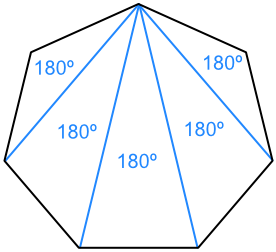Angles in a Polygon

## Angles in a Polygon

A polygon is a shape with straight edges. A shape will either be a regular polygon or an irregular polygon. Regular Polygons have edges that are all of the same length, and angles that all have the same value. Irregular Polygons have at least one angle or length that is different to the others.

Polygons have more than two sides: triangles, rectangles and octagons are all polygons.

The inside angle on a polygon is known as an interior angle. An exterior angle is formed by extending one of the sides, then determining the amount of turn needed to form the new side.An Interior Angle and an Exterior Angle together form a straight line, and therefore add to 180º.

The sum of the exterior angles of a polygon always add up to 360º.The sum of the interior angles can be worked out by first dividing the polygon into triangles:In the example, there are 5 triangles that can be drawn in the 7-sided shape. The sum of the angles is 5 x 180 = 900º.

The sum of the interior angles = (number of sides - 2) x 180

## Example 1

What is the value of the missing angle A in this irregular polygon?The sum of the exterior angles is 360º

360 - 42 - 82 - 78 - 76 = 82º